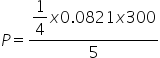Chemistry
Easy

Question

# Calculate the pressure exerted by one gram of He present in a 5L vessel at room temperature.

## 1.224atm12.24atm11.2atmNone of theseHint:

## The correct answer is: 1.224atm

### According to Ideal gas equation, PV = nRT. Here,w = 1 gmV = 5 LT = 300 KHence, we haveP V = w/m RTP x 5 = 1/4 x 0.0821 x 300P = 0.0821 x 15P = 1.23 atm.Hence, (a) 1.224 atm is the correct answer.#### With Turito Foundation.#### Get an Expert Advice From Turito.